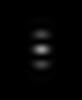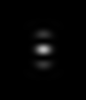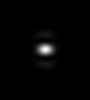## 4pi PSF

Calculated Point Spread Functions for a Four Pi Microscope changing the Numerical Aperture.

"If the two lens segments were full spherical halves, the focal spot would be a (nearly) spherical spot, too. But since a considerable solid angle is not provided by the lenses, interference typically spawns off 2 axial side-lobes which, if not taken into account, lead to artefactual images" (1).

All images are 1 &micro;m height. &alpha; is the angular aperture of the 4pi lens (the total angle of the two segments together as measured from the focus).&alpha; = 194 &deg; </td><td> &alpha; = 212 &deg; </td><td> &alpha; = 252 &deg; </td><td> &alpha; = 288 &deg; </td><td> &alpha; = 352 &deg;

This is a PSF (with some intensity decay in the laterals of the convergent lens due to energy redistribution), not a theoretical 4pi illumination (where intensity would be uniform from all directions of space), but still suggests the real possibility of exciting a central point without noticeable lateral sidelobes.

Microscopic Parameters:

Sample Size: 10 x 10 x 10 nm3